# Calculation register

Calculation registers are the applied configuration objects . They are used in the mechanism of complex periodical calculations and serve to store the records about the specific calculation types that should be executed, as well as to store the intermediate data and the results of executed calculations themselves.

##### Structure

Information in the calculation register is stored as the records each of which contains the values of measures and the corresponding values of resources.

The register measures describe the sections in which the information is stored, and the register resources contain directly the stored information. For example, for the calculation register Main charge of organization employees which has the following structure: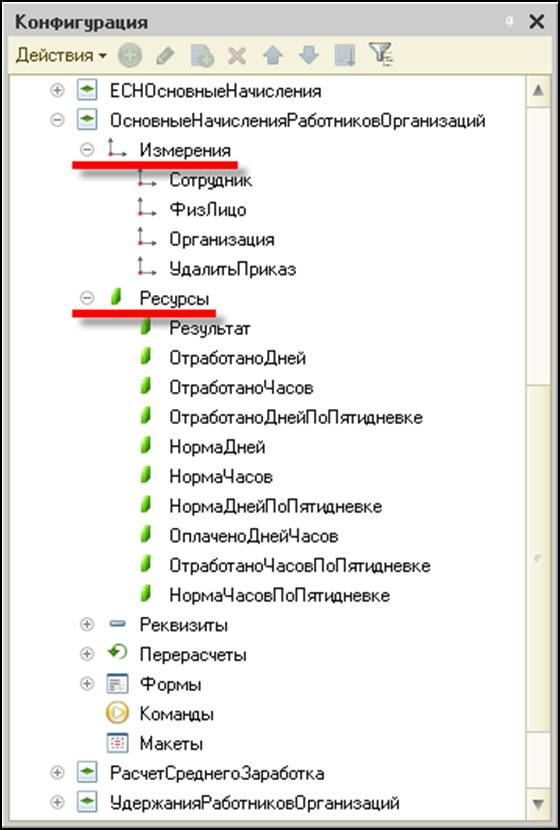the records stored in database will be as follows:##### Relation with chart of calculation types

The calculation register is associated with one of the charts of calculation types existing in the application solution. This relation sets conditions of the presence of field Calculation type for each register record thanks to which the register mechanisms can track the mutual interaction.

##### Periodicity

The calculation register stores the data not only in the section of created measures, but also in the time section. This is the reason of existence of another required field for each register record – Validity period. When creating the calculation register, the developer can indicate the minimal periodicity with which the records will be written in the register:##### Submission to recorder

The change of calculation register state occurs usually during document conduction. Therefore, each register record is connected with a particular document – recorder and with the line number of this document. Adding the records to the register, their changing and deleting is possible only simultaneously for all the records associated with a single document.

##### Relation with timeline

For the calculation register the relationship with timeline can be specified. The timeline is an information register which stores the time scheme of source data involved in the calculations. The measures of this graphic can be, for example, operating schedule and date, and the resource – a number of the hours of service in this date. Then it will be possible to associate the calculation register record with any particular operating schedule and, using the script tools in the sequel, to get information about a number of the hours of service required to execute the calculations.

For example, the timeline which has the following structure: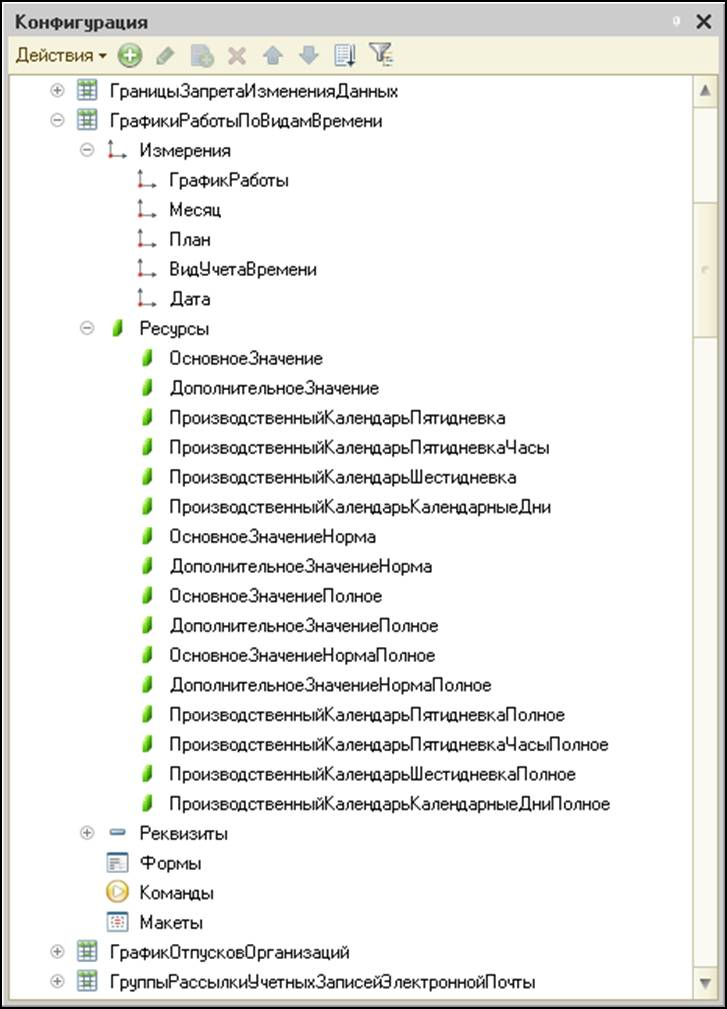may contain information about a number of the hours of service: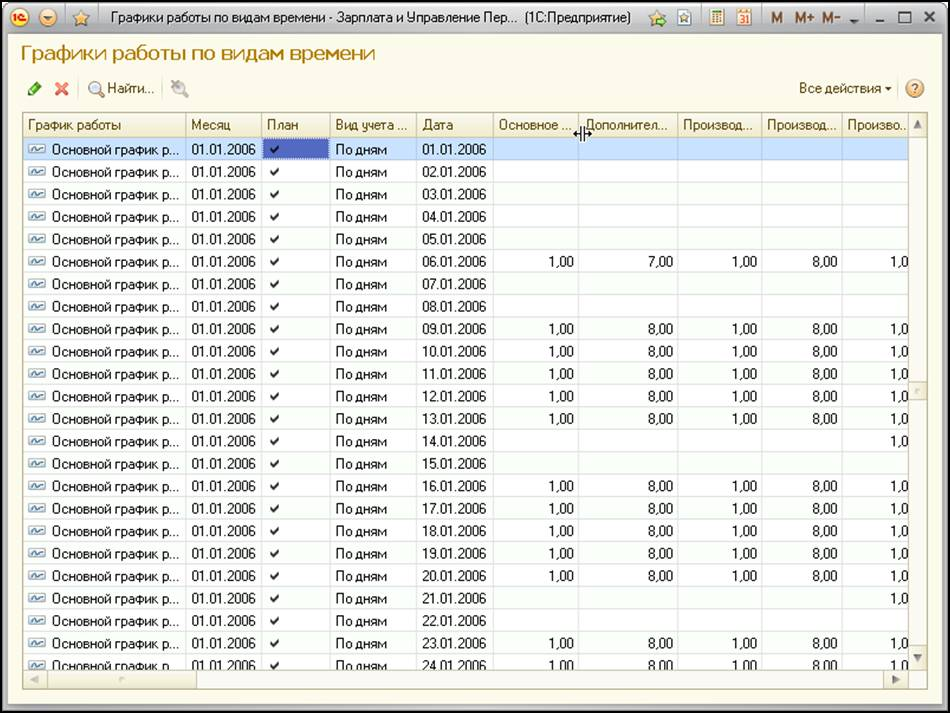##### Re-calculations

The calculation register can include the special objects - Recalculations: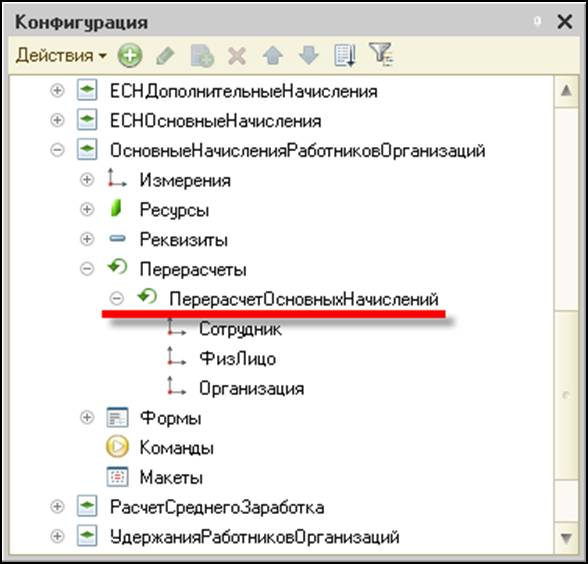In these objects the system will store information about what register records have lost their urgency and are subject to re-calculate as a result of operation of the mechanisms of dependence in accordance with the base period and displacement by validity period.

##### Record uniqueness

The system provides the uniqueness control of the records stored in the calculation register. Therefore, in the calculation register it cannot be two records associated with the same line of the same document.

##### Displacement by validity period

The mechanism of displacement by validity period allows calculating the actual validity period of calculation register record based on the analysis of other records contained in the register.

In general, the calculation register record contains two dates that specify the period on which the operation of this record is covered. This period is called the record validity period. However, if the calculation type to which a given record is related can be displaced with another calculation type, then the validity period of a given record is only a "requested" period, that is, "we want to make record operating in this period". In reality, the actual validity period of this record can be specified only after analyzing all the records of calculation types that displace this calculation type by validity period. The actual validity period will be a set of periods that are the subset of the source validity period of this record. If no record will be found which displaces this one by validity period, then the actual validity period of this record will be equal to its validity period. Another extreme case of displacement by validity period is the complete displacement of a given record with other records. In this case, the actual validity period will be absent.

Each calculation register record contains the calculation type to which it is related. In order to determine which records must displace a given record by validity period, the calculation register uses the relation with a chart of calculation types in which the mutual interaction of calculation types is described. The use of this relation allows the calculation register to determine the actual validity period for every record.

##### Dependence by base period

The mechanism of dependence by base period allows obtaining the value of base for the calculation register record based on the analysis of other records contained in the register.

The base is a numerical value which should be used to calculate the result of a given record. The base is calculated by analyzing the calculation results of other records on which a given record depends by base period. Thus, in general, the calculation register record contains two dates specifying the period in which it is required to analyze the records of calculation types on which a given calculation type depends by base – base period. The use of relation with a chart of calculation types allows the calculation register to determine the calculation types on which a given calculation type depends by base period.

The calculation register supports two types of dependence by base period:

• dependence by validity period;
• dependence by registration period.

In case of dependence by validity period, for obtaining the base only those records will be selected for which the intersection of their actual validity period with a base period of a given record is found. The value of base which is received from a particular influencing record, in general, is not equal to the result which is contained in this record. The base will be calculated in proportion to the fact what part of actual period of influencing record constitutes a section interesting with a specified base period. In this case, the data of graphic associated with this record will be used.

In case of dependence by registration period, for obtaining the base the calculation results will be selected for those records that fall into this record period with the value of its field «Registration period».

The most complex version of dependence is the case when for the calculation type of a given record the property "Validity period is a base period" is set. This property means that as the base period of a given record not base period will be used which is specified in the corresponding record fields, but the actual validity period of the record obtained as a result of operation of the mechanism of displacement by validity period that is, in general, a set of some periods.

##### Forming re-calculation records

The mechanism for forming the recalculation records tracks the fact of occurrence in the register the records that influence on the calculation result of already existing records. The possibility of the influence of new records on the existing ones is defined as a result of analyzing the mutual interaction of calculation types and based on the operation of the mechanisms of displacement by validity period and dependence by base period.

The result of the operation of mechanism to form the recalculation records is a set of recalculation records which contains information about what register records must be calculated over again (recalculated).

##### Calculation register forms

In order to ensure the user to view data contained in the calculation register, the system supports a display form of calculation register – a list form. It allows sorting and selecting the displayed information by several filters: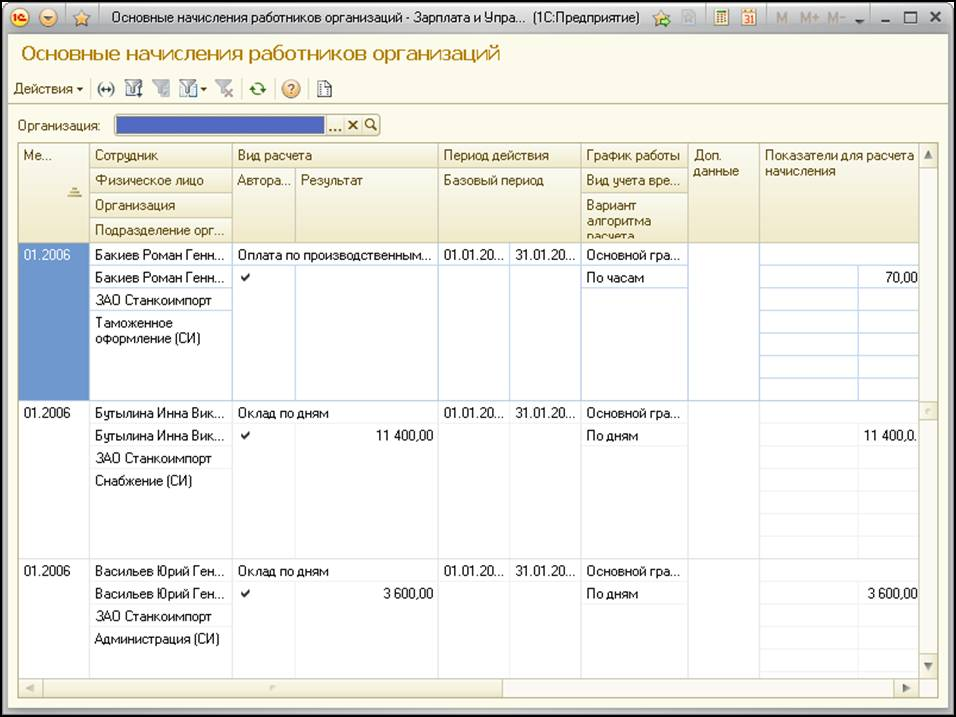The system can automatically generate this form. Along with this, the developer may create his own forms which will be used by the system instead of default forms, including the form of record set which allows adding, changing and deleting the calculation register records.

##### Calculation register functionality

The main functional capabilities that are provided by the calculation register for the developer are:

• selection of records in a given interval by specified filters;
• selection of records by recorder;
• obtaining the base values for the register records that meet the specified selection;
• obtaining the data of graphic for the register records that meet the specified selection;
• obtaining the data of records to be recalculated;
• reading, changing and writing the set of records in the register.